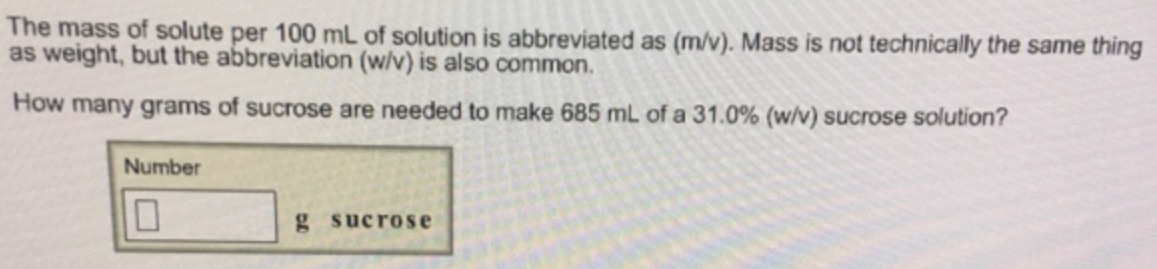# Problem: The mass of solute per 100 mL of solution is abbreviated as (m/v). Mass is not technically the same thing as weight, but the abbreviation (w/v) is also common. How many grams of sucrose are needed to make 685 mL of a 31.0% (w/v) sucrose solution?

###### FREE Expert Solution###### Problem Details

The mass of solute per 100 mL of solution is abbreviated as (m/v). Mass is not technically the same thing as weight, but the abbreviation (w/v) is also common.

How many grams of sucrose are needed to make 685 mL of a 31.0% (w/v) sucrose solution?